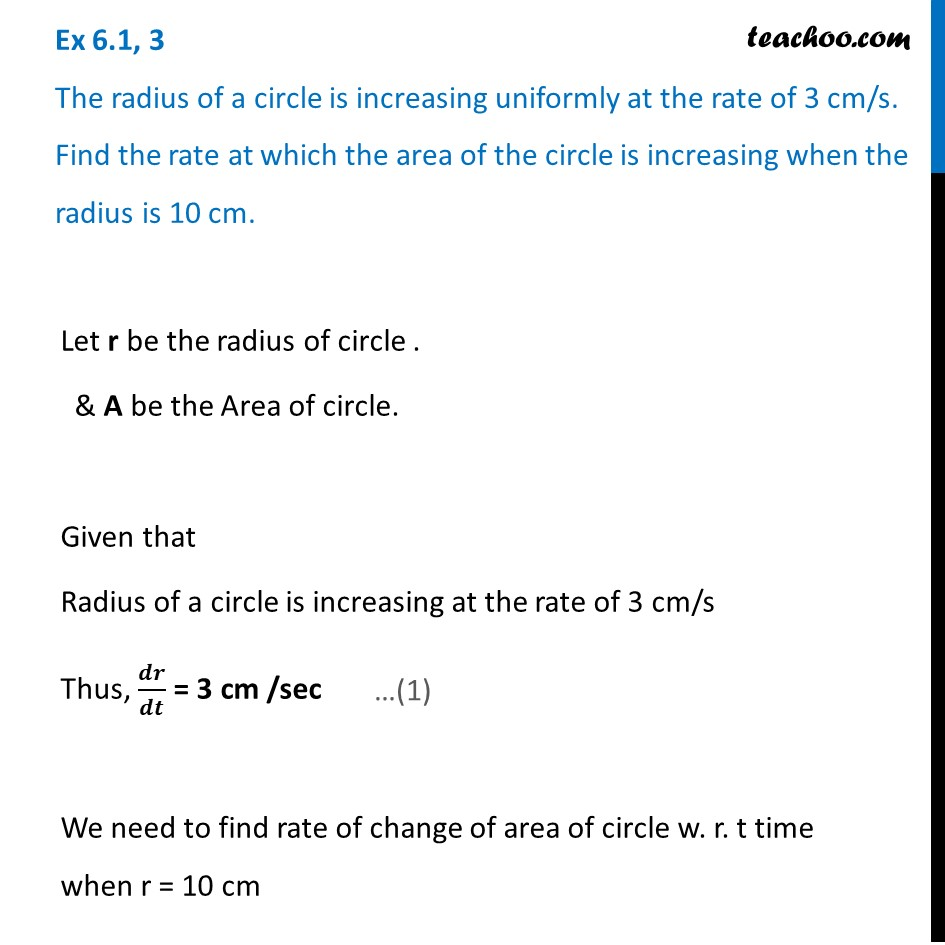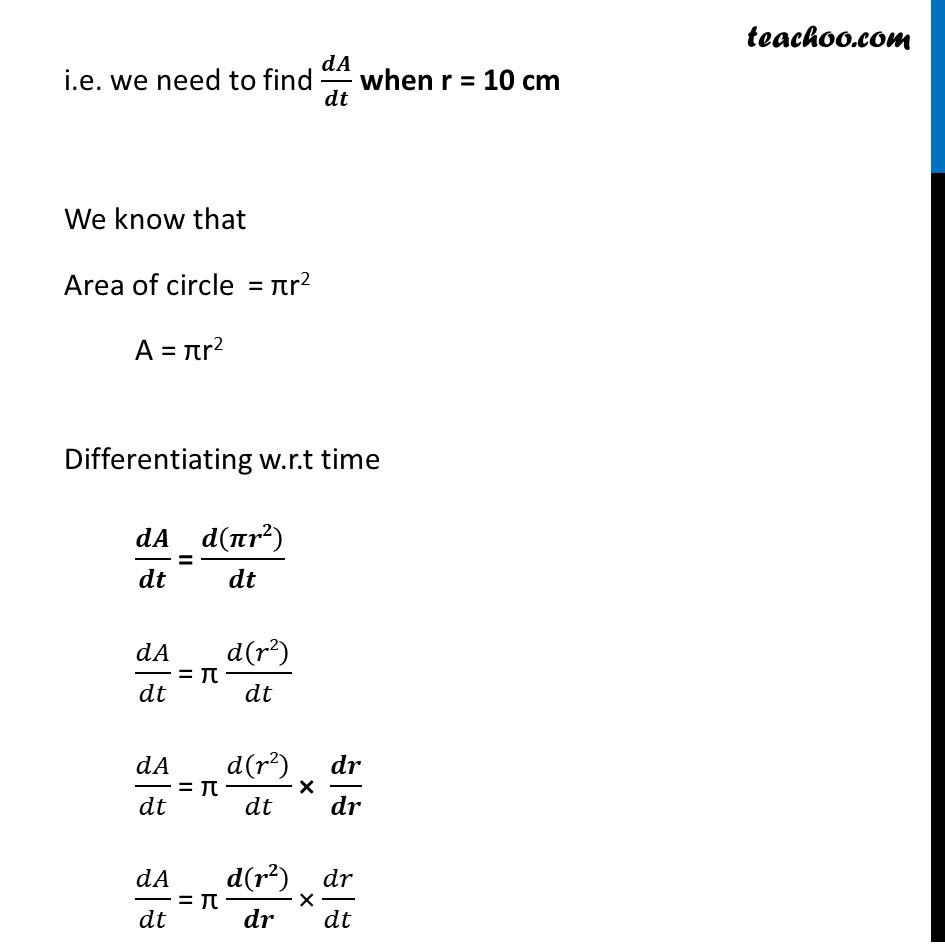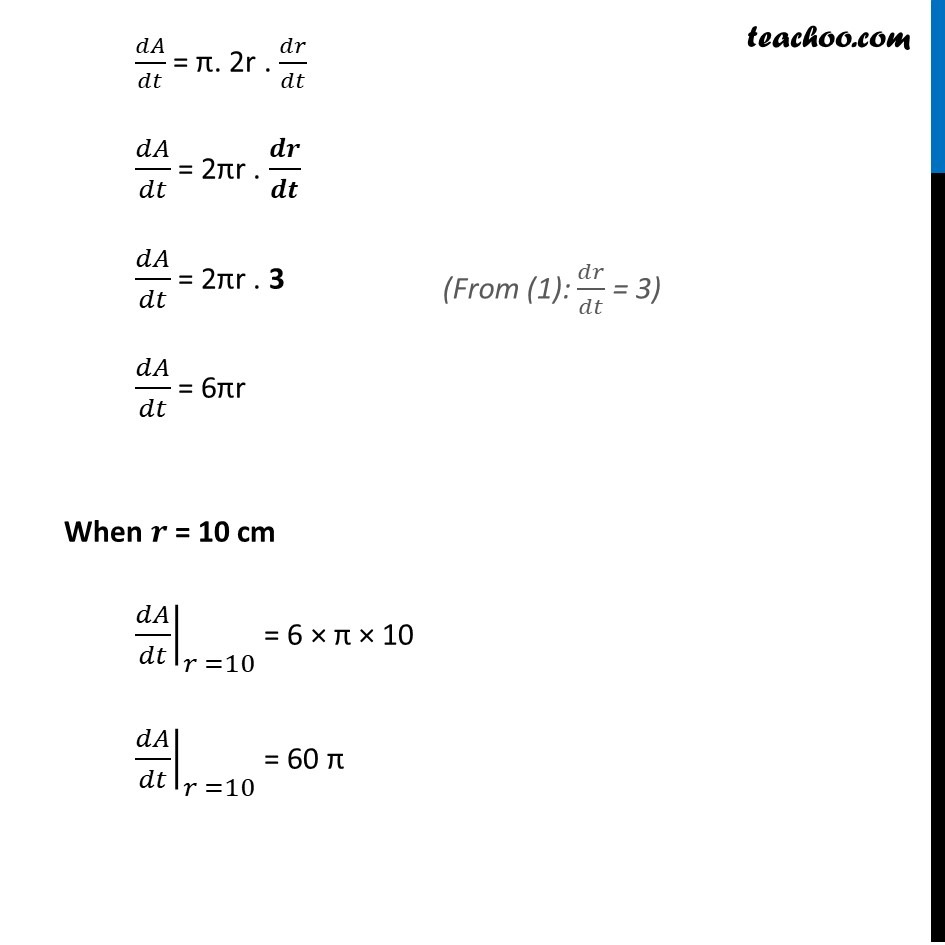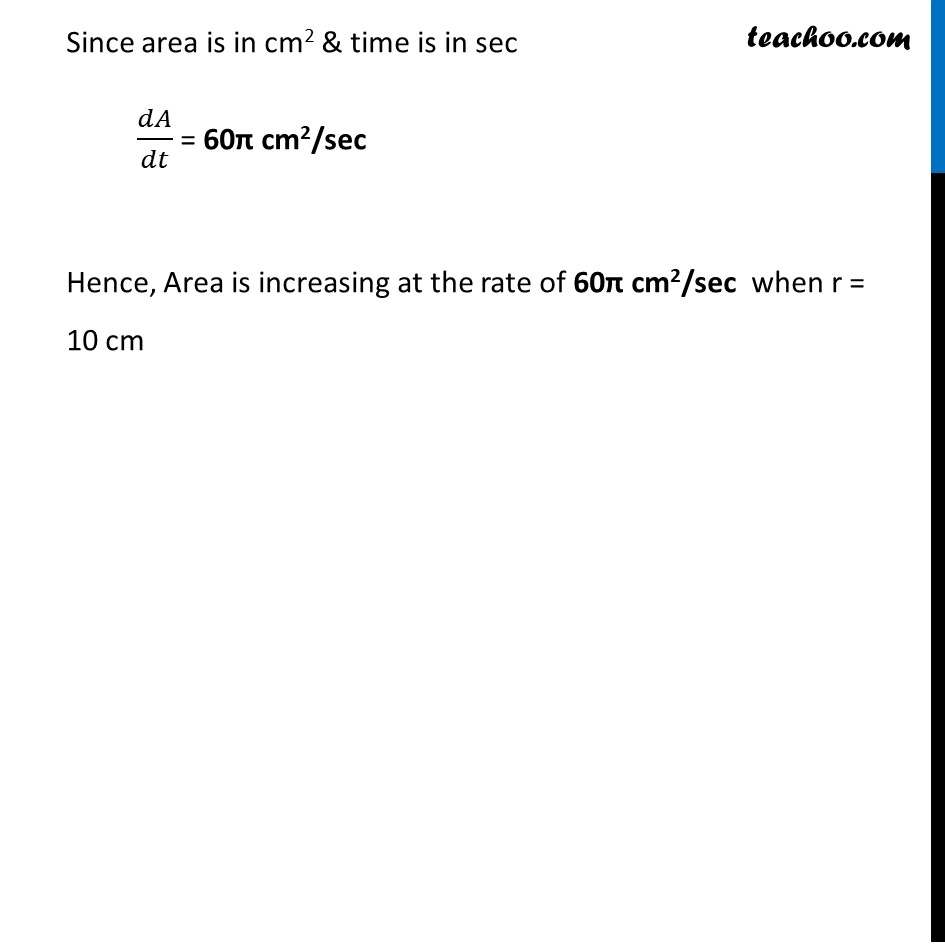1. Chapter 6 Class 12 Application of Derivatives (Term 1)
2. Serial order wise
3. Ex 6.1

Transcript

Ex 6.1, 3 The radius of a circle is increasing uniformly at the rate of 3 cm/s. Find the rate at which the area of the circle is increasing when the radius is 10 cm.Let r be the radius of circle . & A be the Area of circle. Given that Radius of a circle is increasing at the rate of 3 cm/s Thus, 𝒅𝒓/𝒅𝒕 = 3 cm /sec We need to find rate of change of area of circle w. r. t time when r = 10 cm i.e. we need to find 𝒅𝑨/𝒅𝒕 when r = 10 cm We know that Area of circle = πr2 A = πr2 Differentiating w.r.t time 𝒅𝑨/𝒅𝒕 = 𝒅(𝝅𝒓𝟐)/𝒅𝒕 𝑑𝐴/𝑑𝑡 = π 𝑑(𝑟2)/𝑑𝑡 𝑑𝐴/𝑑𝑡 = π 𝑑(𝑟2)/𝑑𝑡 × 𝒅𝒓/𝒅𝒓 𝑑𝐴/𝑑𝑡 = π 𝒅(𝒓𝟐)/𝒅𝒓 × 𝑑𝑟/𝑑𝑡 𝑑𝐴/𝑑𝑡 = π. 2r . 𝑑𝑟/𝑑𝑡 𝑑𝐴/𝑑𝑡 = 2πr . 𝒅𝒓/𝒅𝒕 𝑑𝐴/𝑑𝑡 = 2πr . 3 𝑑𝐴/𝑑𝑡 = 6πr When 𝒓 = 10 cm ├ 𝑑𝐴/𝑑𝑡┤|_(𝑟 =10) = 6 × π × 10 ├ 𝑑𝐴/𝑑𝑡┤|_(𝑟 =10) = 60 π (From (1): 𝑑𝑟/𝑑𝑡 = 3) Since area is in cm2 & time is in sec 𝑑𝐴/𝑑𝑡 = 60π cm2/sec Hence, Area is increasing at the rate of 60π cm2/sec when r = 10 cm